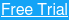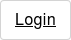## What is a pip in forex trading?

Pip is short for “percentage in point” or “price interest point,” which is the smallest price movement in the exchange rate of a currency pair. For example, the smallest price movement in the currency pair, say, GBP/USD is equal to \$0.0001. Suppose, the price quote of GBP/USD increases from 1.2064 to 1.2068, or \$0.0004, we would say that the GBP gained 4 pips against the U.S dollar.The screenshot above shows the pip digit in the GBP/USD currency pair. Notice that the smallest, right-most digit is called a pipette whereas the fourth digit from the decimal place is called a pip. A pipette is equal to a tenth of a pip.

Furthermore, a pip is equal to 1/100th of one basis point or 1% (1/100 x 1%). For most currency pairs, the fourth digit after the decimal point in price quotes represents a pip. The only exception is the Japanese yen, for which the second digit after the decimal point in the price quote denotes a pip.## How do pips work in forex?

To understand how pips work in forex, consider a currency pair EUR/USD and the relationship between the U.S dollar and euro. In this case, the euro is the base currency while the U.S dollar is the quote currency. For all the currency pairs where the U.S dollar is the quote currency, the pip value would be equal to 0.0001.

## Calculation of Pip Values

In our EUR/USD currency pair example, you can find out the value of one pip by multiplying the lot size or trade value by 0.0001. If you buy a standard lot of euros (100,000 units), the pip value would be \$10 (100,000 x 0.0001). In case you buy a mini lot of euros (10,000 units), the pip value would be \$1 (10,000 x 0.0001).

### Pip Values for USD-denominated accounts

If you have opened a USD-denominated forex account and the USD is mentioned as quote currency in the pair’s price, the pip would always be equal to 0.0001 for all currencies. For example, if you want to find out the value of one pip for GBP/USD currency pair, you need to multiply 0.0001 by a standard lot of 100,000 units of GBP, which calculates to \$10. However, for currency pairs involving the Japanese yen as the quote currency, the pip would be equal to 0.01 because its price is quoted up to 2 decimal places.

However, if the USD in the price quote is the base currency instead of the quote currency, the calculation is slightly different. For example, if the USD/GBP is trading at 0.8266 and you want to calculate its pip value in USD, you will have to divide the pip size by the exchange rate and then multiply the result by the standard lot size of 100,000 units of the base currency USD. The result would be the pip value in USD for a standard lot of USD/GBP.

To illustrate, divide the usual pip value 0.0001 by the USD/GBP exchange rate of 0.8266, which would be calculated as

(0.0001/ 0.8266) = 0.00012097.

Then, multiply the resultant figure (0.00012097) by the standard lot size of 100,000 units to get the pip value for a standard lot:

(0.00012097 x 100,000) = \$12.09.

Alternatively, you can simply divide the pip value for a standard lot (\$10) by the exchange rate (0.8299) to determine the pip value for a standard lot:

(\$10/0.8299) = \$12.09 per pip for a standard lot.

### Pip Values for non-USD denominated accounts

If you have opened an account in a non-US currency, say GBP, and your account currency is mentioned as quote currency in the price quote, calculating the pip value would simply be the minimum price movement in the currency pair. For example, if you want to determine the pip value for a standard lot of USD/GBP, the pip value would be 10 GBPs for a standard lot, 1 GBP for a mini lot, and 0.10 GBP for a micro lot.

However, if your account currency, say GBP, is the base currency instead of the quote currency in a currency pair, you need to divide the pip size by the exchange rate and then multiply the result by the lot size of your account currency. The calculation for it is the same as we demonstrated in our USD/GBP example. Suppose, the GBP/USD is trading at 1.2098, you can divide the pip size, 0.0001 by the exchange rate (1.2098) and then multiply the result by the standard lot (100,000 units of base currency). Here are the calculations:

(0.0001/ 1.2098) x 100,000 units = GBP 8.26 per pip for a standard lot

## Pips examples:

Taking our GBP/USD example, if you buy one standard lot of GBP and the exchange rate rises from 1.2098 to 1.2100, you would gain 2 pips on the trade. If you sell the standard lot (100,000 units) of GBP at 1.2100, you would make a profit of 2 pips, or GBP 16.52 (GBP 8.26 per pip x 2 pips).

Let’s consider another example:

Suppose, you buy one standard lot of EUR/USD with the expectation that the euros would appreciate against the USD, allowing you to profit from the trade. If the exchange rate rises from 1.0426 to 1.0432, you would gain 6 pips on the trade. If your account is USD-denominated, your gain on the trade would be \$60 (\$10 x 6 pips).

## How much are 50 pips worth?

In US dollar terms, fifty pips are worth \$500 for one standard lot, \$50 for a mini lot, and \$5 for a micro lot. Here are the detailed calculations for different lot sizes:

50 pips’ worth in 1 standard lot = minimum pip size x number of units in a lot x number of pips
= \$0.0001 x 100,000 x 50
= \$500

50 pips’ worth in 1 mini lot = minimum pip size x number of units in a lot x number of pips
= \$0.0001 x 10,000 x 50
= \$50

50 pips’ worth in 1 micro lot = minimum pip size x number of units in a lot x number of pips
= \$0.0001 x 1000 x 50
= \$5

## How much is \$1 in pips?

One pip is worth \$1 for a mini lot, which means that if you buy 10,000 units or a mini lot of US dollars, one pip change in the price quote would equal \$1. In short, \$1 equals one pip if you trade a mini lot of US dollars.

## How many dollars are in 100 pips?

One hundred pips in US dollar terms are worth \$1,000 for one standard lot, \$100 for a mini lot, and \$10 for a micro lot. Here are the detailed calculations for different lot sizes:

U.S dollars in 100 pips in 1 standard lot = minimum pip size x number of units in a lot x number of pips
= \$0.0001 x 100,000 x 100
= \$1,000

50 pips’ worth in 1 mini lot = minimum pip size x number of units in a lot x number of pips
= \$0.0001 x 10,000 x 100
= \$100

50 pips’ worth in 1 micro lot = minimum pip size x number of units in a lot x number of pips
= \$0.0001 x 1000 x 100
= \$10

## How many pips is a good trade?

Because financial markets move in an unpredictable fashion, one cannot specify in absolute terms how many pips a trader should aim to get or how many pips’ gain is a good trade. There could be times when you can make 20, 30, 50, or even 100 pips gains, while there could be times when you book losses of similar pips as well.

You should aim to take only those trades where you have a chance to earn three times the pips you are risking on your trade. Some currency pairs move by more than 100 pips per day, and you can capitalize on these movements, depending on your strategy and market conditions. As always, we recommend employing a good risk management strategy while trading any asset class or commodity.

## How many pips does the average retail forex trader make?

While there is no empirical data that confirms how many pips an average retail forex trader makes. However, we can estimate, based on forex-related data, such as average pips movement in a forex pair per day, percentage of successful traders over the long term, and other similar metrics. For example, some forex pairs move 100 pips per day on average, allowing traders to profit from the movement. If a trader even makes 10 pips per day daily, it can result in significant profit, based on the number of lots traded.

This will all depend upon your strategy, discipline and experience level in trading forex pairs and understanding trading strategies#### History of Bear Markets

As we find ourselves in the midst of a brutal bear market in 2022, it may be a good exercise to study the past in order to be better educated on what...

## What is a Bear Market?

How exactly is a bear market defined? The most commonly used definition for a bear market is when major indices, like the S&P...